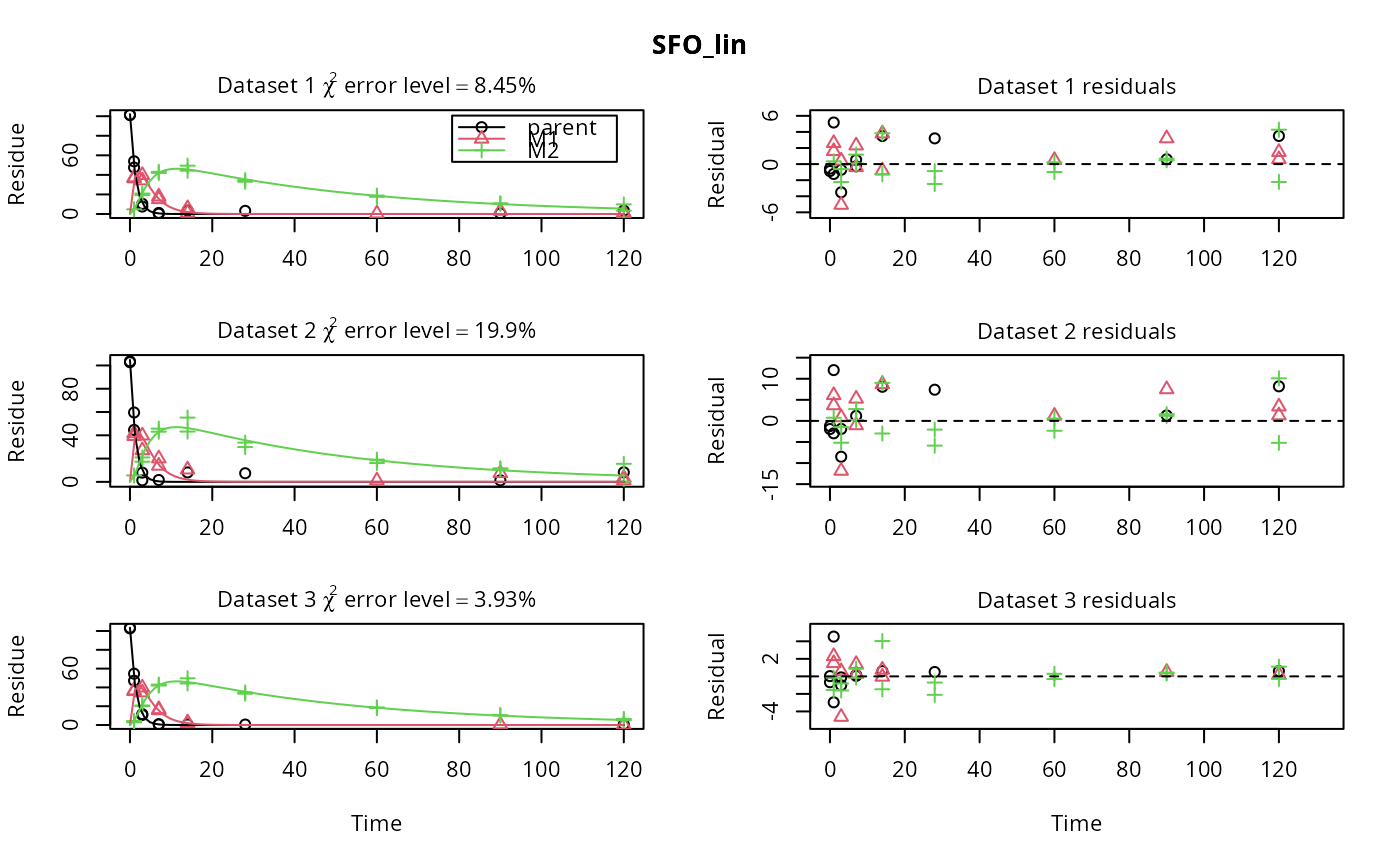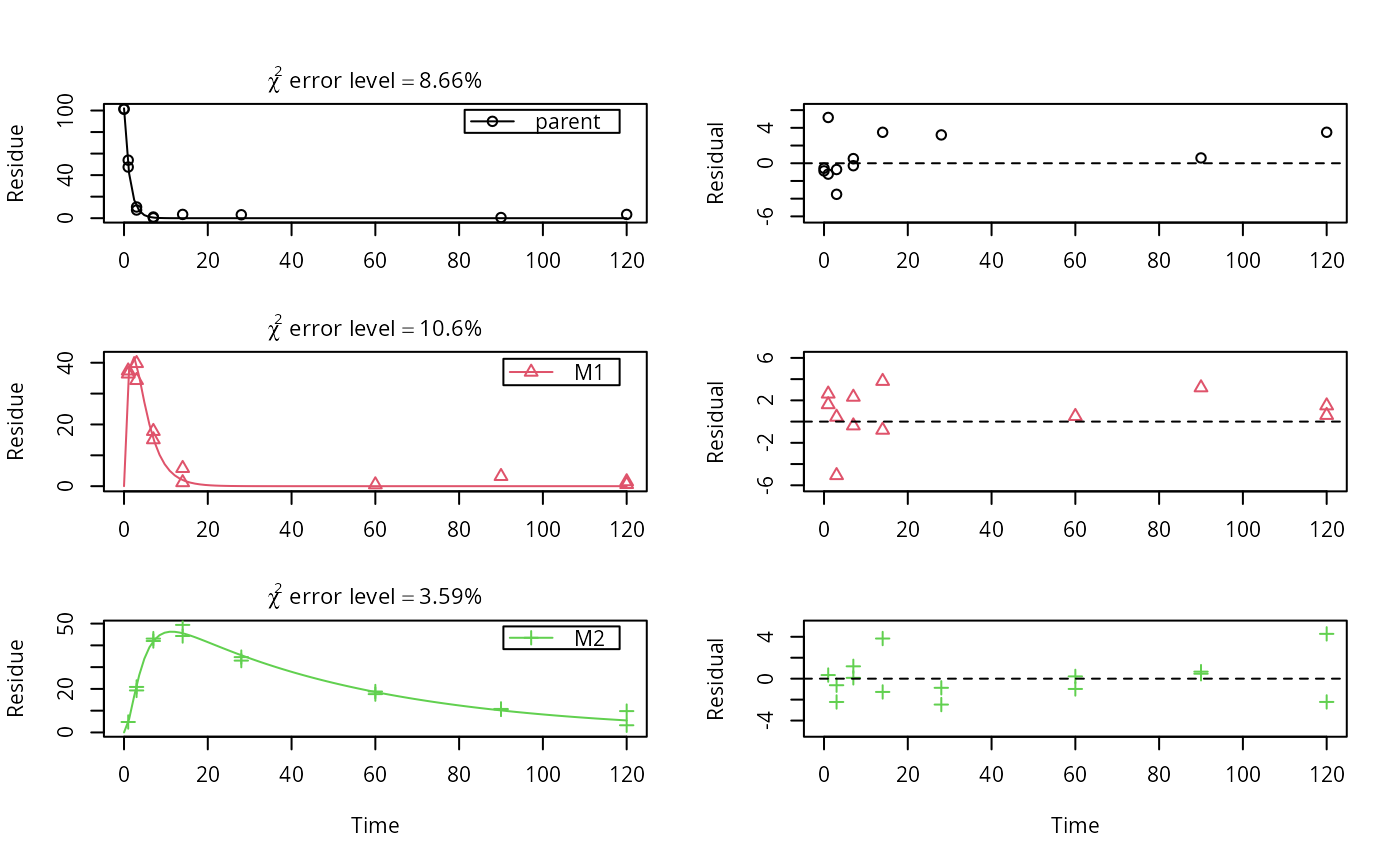This function calls mkinfit on all combinations of models and datasets specified in its first two arguments.

mmkin(
models = c("SFO", "FOMC", "DFOP"),
datasets,
cores = detectCores(),
cluster = NULL,
...
)

## Arguments

models Either a character vector of shorthand names like c("SFO", "FOMC", "DFOP", "HS", "SFORB"), or an optionally named list of mkinmod objects. An optionally named list of datasets suitable as observed data for mkinfit. The number of cores to be used for multicore processing. This is only used when the cluster argument is NULL. On Windows machines, cores > 1 is not supported, you need to use the cluster argument to use multiple logical processors. Per default, all cores detected by parallel::detectCores() are used. A cluster as returned by makeCluster to be used for parallel execution. Further arguments that will be passed to mkinfit.

## Value

A two-dimensional array of mkinfit objects and/or try-errors that can be indexed using the model names for the first index (row index) and the dataset names for the second index (column index).

[.mmkin for subsetting, plot.mmkin for plotting.

Johannes Ranke

## Examples


# \dontrun{
m_synth_SFO_lin <- mkinmod(parent = mkinsub("SFO", "M1"),
M1 = mkinsub("SFO", "M2"),
M2 = mkinsub("SFO"), use_of_ff = "max")
#> Successfully compiled differential equation model from auto-generated C code.
m_synth_FOMC_lin <- mkinmod(parent = mkinsub("FOMC", "M1"),
M1 = mkinsub("SFO", "M2"),
M2 = mkinsub("SFO"), use_of_ff = "max")
#> Successfully compiled differential equation model from auto-generated C code.
models <- list(SFO_lin = m_synth_SFO_lin, FOMC_lin = m_synth_FOMC_lin)
datasets <- lapply(synthetic_data_for_UBA_2014[1:3], function(x) x$data) names(datasets) <- paste("Dataset", 1:3) time_default <- system.time(fits.0 <- mmkin(models, datasets, quiet = TRUE)) time_1 <- system.time(fits.4 <- mmkin(models, datasets, cores = 1, quiet = TRUE)) #> Warning: Optimisation did not converge: #> false convergence (8)#> Warning: Shapiro-Wilk test for standardized residuals: p = 0.0117#> Warning: Shapiro-Wilk test for standardized residuals: p = 0.0174 time_default #> user system elapsed #> 4.828 0.413 1.467 time_1 #> user system elapsed #> 5.228 0.000 5.231 endpoints(fits.0[["SFO_lin", 2]]) #>$ff
#>   parent_M1 parent_sink       M1_M2     M1_sink
#>   0.7340478   0.2659522   0.7505691   0.2494309
#>
#> \$distimes
#>              DT50       DT90
#> parent  0.8777688   2.915885
#> M1      2.3257466   7.725963
#> M2     33.7200800 112.015681
#>
# plot.mkinfit handles rows or columns of mmkin result objects
plot(fits.0[1, ])plot(fits.0[1, ], obs_var = c("M1", "M2"))plot(fits.0[, 1])# Use double brackets to extract a single mkinfit object, which will be plotted
# by plot.mkinfit and can be plotted using plot_sep
plot(fits.0[[1, 1]], sep_obs = TRUE, show_residuals = TRUE, show_errmin = TRUE)plot_sep(fits.0[[1, 1]])
# Plotting with mmkin (single brackets, extracting an mmkin object) does not
# allow to plot the observed variables separately
plot(fits.0[1, 1])# }